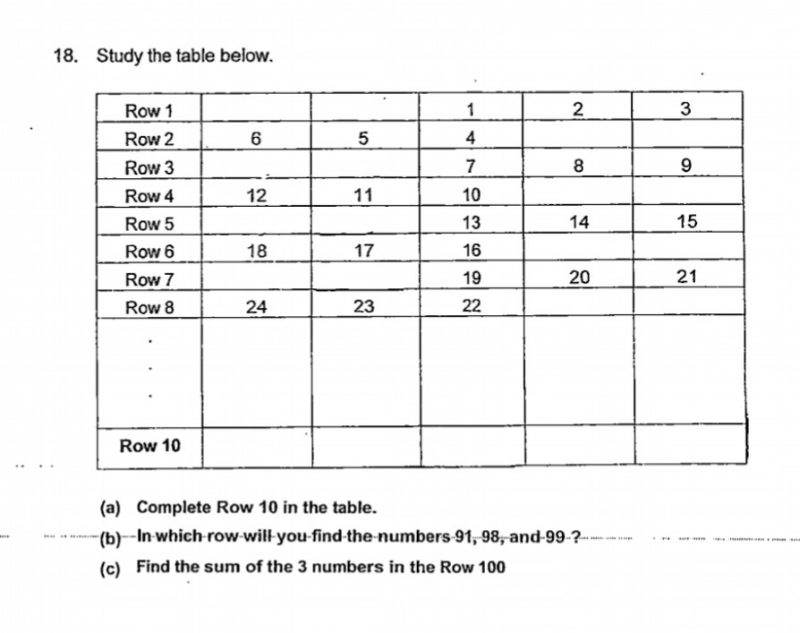# Questionhi, can help with this qn parts (b) and (c). tq!

Source: Tao Nan

(b)  I think there is an error in the question.

Every row has three consecutive numbers. To determine the row number, divide the number by 3 and round up if there is a fraction in the answer.

91 appears in Row 31 (91/3 = 30 1/3, round up to 31)

97, 98 and 99 appear in Row 33 (97/3 = 32 1/3, round up to 33).

(c)  100 x 3 = 300

The last number in Row 100 is 300. The numbers are 298, 299 and 300.

298 + 299 + 300 = 897

0 Replies 0 Likes